#Script-based Visualization
MRST supports three basic, but quite flexible, routines for visualisation of grids and face and cell data, as well as a few helper routines that e.g., outline coarse grids and plot well paths. More advanced visualisations can be made by combining the basic routines and standard MATLAB functionality, e.g., as shown below. For special grid types, MRST also supports tracing of streamlines using Pollock's method.

### Plotting Cell Data

MRST provides the basic routine plotCellData for visualisation of cell data. The routine assumes that one scalar value is associated with each cell in a grid structure and visualises the scalar data by plotting all exterior grid faces, colored by the given data values. The graphical output can be restricted to a subset of the grid by specifying a vector of cell numbers to be visualised. The visualisation is implemented in terms of the low-level MATLAB function PATCH, which means that all properties supported by PATCH can be passed as argument to plotCellData. The figures below show a few examples of simple visualisations obtained using plotCellData. MRST also provides the routine plotGrid, which is a special case of plotCellData.

### Plotting Face Data

MRST provides the basic routine plotFaceData for visualisation of face data. The routine assumes that one scalar value is associated with each face in a grid structure and visualises the scalar data by plotting all exterior grid faces, colored by the given data values. The graphical output can be restricted to a subset of the grid by specifying a vector of cell numbers to be visualised. The visualisation is implemented in terms of the low-level MATLAB function PATCH, which means that all properties supported by PATCH can be passed as argument to plotFaceData.

### Examples

The figures below show several examples of how one can combine different features of the two basic routines plotCellData and plotFaceData to create different plots. We also illustrate some of the special-purpose routines that are supplied with MRST.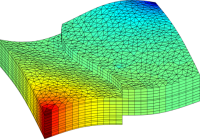Solution of a quarter five-spot problem on a prismatic grid with a single curved fault, visualised by a straightforward call plotCellData(G,p).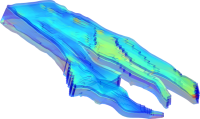Visualisation of the face area for a model from the Norwegian Sea using transparent colors (setting FaceAlpha) and no coloring of the grid lines (setting EdgeColor to 'none').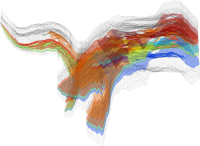Visualisation of semi-impermeable layers in a model from the Norwegian Sea, plotted using transparent colors (setting FaceAlpha in plotFaces) and the grid outlined using no face colors.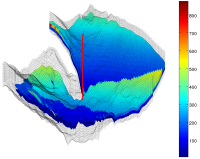Visualisation of the high-permeable parts of the Johansen formation. The grid is outlined using plotGrid with no surface colors and a potential injection well is plotted using plotWell.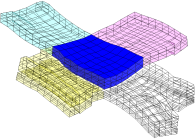Close examination of a coarse block using plotBlockAndNeighbors, which plots a coarse block and its neighbors using different colors and levels of transparency .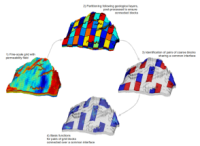Illustration of key steps in a multscale pressure solver. The permeability is plotted using plotCellData, the partition is illustrated by plotting the partition vector with plotCellData and adding outlineCoarseGrid, whereas the basis functions in the last two plots are plotted by multiple calls to plotCellData.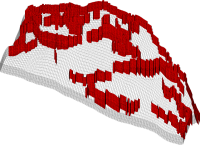Visualisation of a structural model from the SAIGUP study. The grid is outlined using the plotGrid routine with the FaceColor property set to 'none', whereas fault faces are plotted using plotFaces.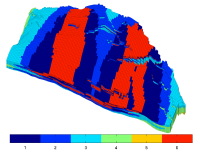Visualisation of different rock types from one of the petrophysical realisations in SAIGUP. The geological model represents a shallow-marine reservoir.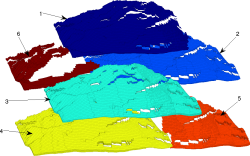Visualisation of different rock types for a SAIGUP model; plotCellData was called six times with the visible cells set to one of the rock types and with different translations of the grid.

Published October 19, 2010